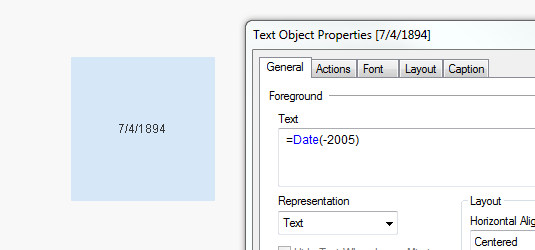New to Qlik Sense

Discussion board where members can get started with Qlik Sense.

New Contributor III

Variable's returning wrong values

Hi,

I am using two variables to show two separate pieces of data. However, they are both returning the wrong (although similarish) values.

I have defined by variables in the variable editor in Sense as:

v_LatestCLD =  max ( { \$<[Timeline Content]={"CLD"}> } [Timeline Start Date] )

v_PreviousCLD = max( { <[Timeline Content] = {'.'}> * < [Timeline Date Change] =   {'<=\$(=\$(vTest1))'} > } [Timeline Start Date] )

Where vTest1 is a variable that is attached to an input box, hence why these statements (at least the 2nd one) cannot be loaded in the load script. I have two tables showing the data, one that displays the above formula in measure columns, the second which displays the values calculated using variables, using the below forumla

date(\$(v_PreviousCLD),'DD-MM-YYYY'

date(\$(v_CurrentCLD),'DD-MM-YYYY'

Table 1:     14-01-2018     31-12-2018

Table 2:     04-07-1894     10-07-1894

What am I doing wrong?

FYI, the requirement for the final state is to only display values in the table in which the dates are different. If v_PreviousCLD = v_CurrentCLD, that row should not be displayed.

3 RepliesMVP

Re: Variable's returning wrong values

Try without dollar sign expansions

Date(v_PreviousCLD, 'DD-MM-YYYY')

Date(v_CurrentCLD, 'DD-MM-YYYY')MVP

Re: Variable's returning wrong values

Dollar sign expansion might be evaluating the Date as a calculation

14-1-2018 = -2005 converted to date might be 04-07-1894MVP

Re: Variable's returning wrong values

Look at this